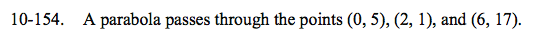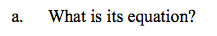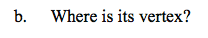Home > CCA2 > Chapter Ch10 > Lesson 10.3.1 > Problem10-154

10-154.
1. A parabola passes through the points (0, 5), (2, 1), and (6, 17). Homework Help ✎

1. What is its equation?

2. Where is its vertex?Substitute each point into the equation y = ax2 + bx + c.
You will have a system of three equations with three unknowns.
One of your equations should be 1 = 4a + 2b + c.$x=\frac{-b}{2a}$